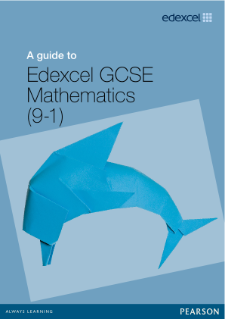# Mathematics

Key Stage 3

Students will develop their understanding of a range of mathematical concepts, methods and vocabulary, studying topics such as number, algebra, ratio, proportion, geometry, measures, probability and statistics. Students will be equipped with the ability to mathematically describe, analyse and reason and to think independently in applied and abstract ways. Students will build a solid foundation in preparation for Key Stage 4, as well as develop their confidence in Mathematics.

 YEAR 7 TERM 1 Analysing and displaying data  Number Skills  Equations, functions and formulae  Fractions TERM 2 Lines, angles and shapes  Decimals and measures  Equations  Probability  Ratio and proportion TERM 3 Multiplicative reasoning  Perimeter, area and volume  Sequences and graphs  Transformations

 YEAR 8 TERM 1 Number  Factors and powers  2D shapes and 3D solids  Real life graphs TERM 2 Transformations  Fractions, decimals and percentages  Constructions and loci  Decimal and ratio TERM 3 Probability  Scale drawings and measures  Graphs

 YEAR 9 TERM 1 Powers and roots  Quadratics  Inequalities, equations and formulae  Standard form  Collecting and analysing data TERM 2 Multiplicative reasoning  Non-linear graphs  Accuracy and measures  Constructions  Circle, Pythagoras and prisms TERM 3 Graphical solutions  Trigonometry  Mathematical reasoning  Probability  Comparing shapes

GCSE Mathematics

All students study Mathematics at GCSE.

Students will expand on their existing knowledge and learn about the various areas of mathematics, such as number, algebra, geometry, ratio and proportion, probability and statistics. Students will logically reason to draw conclusions from mathematical information and communicate their ideas using mathematical arguments and vocabulary.  They will also develop their problem solving and the ability to make connections, interpret and evaluate. Students will also appreciate the extensive part that Mathematics plays in daily life, preparing them well for the future.

 YEAR 10 TERM 1 Number  Algebra  Graphs, tables and charts  Fractions and percentages  Equations, inequalities and sequences TERM 2 Angles  Averages and range  Perimeter, area and volume 1  Graphs  Transformations TERM 3 Ratio and proportion  Right-angled triangles  Probability  Multiplicative reasoning  Constructions, loci and bearings

 YEAR 11 TERM 1 Quadratic equations and graphs  Perimeter, area and volume 2  Fractions, indices and standard form TERM 2 Congruence and similarity  Vectors  More algebra TERM 3 Final exam preparation

For more detailed information on the course and assessment, please visit